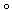## Homework Statement

1. Find a formula for
(fg)(x) = ?

2. Find a formula for
(ff )(x) = ?

3. Find a formula for the composition below.
g(h(x)) =

4. Find a formula for the composition below.
(hg)(x) =

## The Attempt at a Solution

1. f(g(x))
2. f(f(x))
3. (g º h)(x)
4. h(g(x))

Why are these incorrect?

Mark44
Mentor

## Homework Statement

1. Find a formula for
(fg)(x) = ?

2. Find a formula for
(ff )(x) = ?

3. Find a formula for the composition below.
g(h(x)) =

4. Find a formula for the composition below.
(hg)(x) =

## The Attempt at a Solution

1. f(g(x))
2. f(f(x))
3. (g º h)(x)
4. h(g(x))

Why are these incorrect?
All you have done is to rewrite each composition in a different form. I suspect that there are formulas for f, g, and h that you have overlooked.